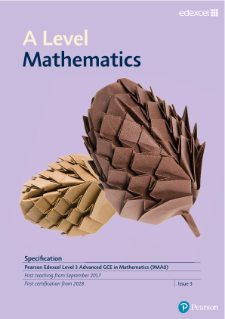# A LEVEL MATHEMATICS

Students will extend their learning from KS4 to A Level by exploring applications of mathematics via the route of Statistics and Mechanics alongside Pure Mathematics. Statistics is the collection and analysis of data, using this to make predictions of future events. Mechanics is the analysis of the physical world around us, including the study of forces and motion. The course is highly challenging and interesting, honing students’ ability to think logically and analytically as well as problem solve.

 YEAR 12 TERM 1 Pure  Algebra and functions  Coordinate geometry  Further algebra  Trigonometry  Differentiation TERM 2 Pure  Vectors (2D)  Integration    Applied  Quantities and units in mechanics (Mechanics)  Kinematics (Mechanics)  Forces and Newton’s Laws (Mechanics)  Statistical sampling (Statistics)  Data presentation and interpretation (Statistics)  Probability (Statistics)  Statistical Distribution (Statistics) TERM 3 Pure  Exponentials and logarithms    Applied  Further Kinematics (Mechanics)  Statistical Hypothesis Testing (Statistics)    Pure (A2)  Proofs  Algebraic and partial fractions  Functions and modelling    Revision  Assessments

 YEAR 13 TERM 1 Pure  Sequenced and Series  The binomial theorem  Trigonometry  Parametric equations  Differentiation    Applied  Regression and correlation (Statistics) TERM 2 Pure  Numerical methods  Integration    Applied  Projectiles (Mechanics)  Moments (Mechanics)  Forces and any angle (Mechanics)  Applications of kinematics (Mechanics)  Applications of forces (Mechanics)  Regression and correlation Probability (Statistics)  The Normal Distribution (Statistics) TERM 3 Pure  Vectors    Applied  The Normal Distribution cont. (Statistics)  Revision and preparation for A Level examinations

For more detailed information click on the icon below: